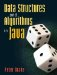# A.3. Loops

Loops allow us to write a program which does something many times in a row with only a few lines of code. There are three ways to write loops in Java. We can always use any one of them, but there are circumstances in which one is clearer than another.

The first loop is the while loop (Figure A-5). This consists of the keyword while, a test (boolean expression) in parentheses, and then a body in curly braces. Java first evaluates the test expression. If it evaluates to true, the body of the loop is then executed. The test is then evaluated again, and so on.

Figure A-5. Saying hello to each person named on the command line using a while loop.

 ```1 /** Print a friendly greeting. */ 2 public static void main(String[] args) { 3 int index = 0; 4 while (index < args.length) { 5 System.out.println("Hello, " + args[index] + "!"); 6 index = index + 1; 7 } 8 } ```

Very similar to the while loop is the do loop (Figure A-6). This is almost the same, except that the body of the loop is executed once before the test is evaluated. For this program, the do loop actually introduces a subtle bug, which we will fix in Section A.5.

Figure A-6. This do loop is not exactly equivalent to the while loop in Figure A-5. It guarantees that the body of the loop is executed at least once.

 ```1 /** Print a friendly greeting. */ 2 public static void main(String[] args) { 3 int index = 0; 4 do { 5 System.out.println("Hello, " + args[index] + "!"); 6 index = index + 1; 7 } while (index < args.length); 8 } ```

Finally, there is the for loop (Figure A-7). While a bit cryptic, it allows for a very concise statement of the loop. It consists of the keyword for, a loop header in parentheses, and then a body in curly braces. The loop header has three parts, separated by semicolons: an initialization statement, a test, and an update. Java first evaluates the initialization statement and then the test. If the test is false, the loop ends. Otherwise, the body and the update are both executed, the test is evaluated again, and so on.

Figure A-7. This for loop is exactly equivalent to the while loop in Figure A-5.

 ```1 /** Print a friendly greeting. */ 2 public static void main(String[] args) { 3 int index; 4 for (index = 0; index < args.length; index = index + 1) { 5 System.out.println("Hello, " + args[index] + "!"); 6 } 7 } ```

For loops like this are extremely common. Programmers typically take advantage of a few shortcuts to make the loop even more concise:

• The loop variable can be declared as part of the initialization statement. If this is done, the variable disappears as soon as the loop ends.
• If the loop variable is an index into an array, it is almost invariably named i.
• The loop variable can be incremented with the shorter statement i++.

The resulting method (Figure A-8) behaves exactly like the previous version.

Figure A-8. Cosmetic changes produce a more typical-looking for loop.

 ```1 /** Print a friendly greeting. */ 2 public static void main(String[] args) { 3 for (int i = 0; i < args.length; i++) { 4 System.out.println("Hello, " + args[i] + "!"); 5 } 6 } ```

Java 1.5 introduces an enhanced for loop which allows us to loop through the elements of an array in an even more concise manner. In Figure A-9, line 3 can be read, "For each String name in args...." On the first pass through the loop, name is short for args. On the next pass, name is short for args, and so on.

Figure A-9. The enhanced for loop in Java 1.5 makes looping through an array even easier.

 ```1 /** Print a friendly greeting. */ 2 public static void main(String[] args) { 3 for (String name : args) { 4 System.out.println("Hello, " + name + "!"); 5 } 6 } ```

The enhanced for loop can also be used on iterable data structures (Section 5.4).

Exercises

 A.8 What is the scope of index in Figure A-7? What is the scope of i in Figure A-8? (Hint: Try printing the variable after the loop ends.) A.9 Using each kind of loop, print the numbers 1 through 10. A.10 Using two nested for loops, print a multiplication table for the numbers 1 through 10.Data Structures and Algorithms in Java
ISBN: 0131469142
EAN: 2147483647
Year: 2004
Pages: 216
Authors: Peter Drake